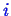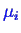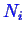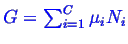Next: Chemical Reaction Equilibria Up: Continuum Thermodynamics Previous: Minimum Principles

## Gibbs Free Energy is Minimized at constant Pressure and Temperature

The Gibbs free energy is partitioned into a potential for each chemical species,and the number of moles of,. Then, the Gibbs free energy,must be a minimum. Knowing that it is a minimum means its derivative is zero--and this gives us a bunch of useful equations that apply to states of equilibrium. The fact that it is a minimum also means that its second derivative is positive definite--this implies restrictions on the properties of stable materials (such as the bulk modulus and the heat capacity must be positive).

W. Craig Carter 2002-09-05